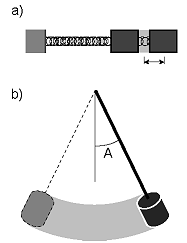Lectures on Physics has been derived from Benjamin Crowell's Light and Matter series of free introductory textbooks on physics. See the editorial for more information....# Period, Frequency, and AmplitudeThe figure shows our most basic example of a vibration. With no forces on it, the spring assumes its equilibrium length, (a). It can be stretched, (b), or compressed, (c). We attach the spring to a wall on the left and to a mass on the right. If we now hit the mass with a hammer, (d), it oscillates as shown in the series of snapshots, (d)-(m). If we assume that the mass slides back and forth without friction and that the motion is one-dimensional, then conservation of energy proves that the motion must be repetitive. When the block comes back to its initial position again, (g), its potential energy is the same again, so it must have the same kinetic energy again. The motion is in the opposite direction, however. Finally, at (j), it returns to its initial position with the same kinetic energy and the same direction of motion. The motion has gone through one complete cycle, and will now repeat forever in the absence of friction.

The usual physics terminology for motion that repeats itself over and over is periodic motion, and the time required for one repetition is called the period, T (the symbol P is not used because of the possible confusion with momentum). One complete repetition of the motion is called a cycle.

We are used to referring to short-period sound vibrations as "high" in pitch, and it sounds odd to have to say that high pitches have low periods. It is therefore more common to discuss the rapidity of a vibration in terms of the number of vibrations per second, a quantity called the frequency, f. Since the period is the number of seconds per cycle and the frequency is the number of cycles per second, they are reciprocals of each other,

f = 1/T .A carnival game.Period and frequency of a fly's wing-beats.

Units of inverse second, s-1, are awkward in speech, so an abbreviation has been created. One Hertz, named in honor of a pioneer of radio technology, is one cycle per second. In abbreviated form, 1 Hz=1 s-1. This is the familiar unit used for the frequencies on the radio dial.Frequency of a radio station.

Units of frequency are also commonly used to specify the speeds of computers. The idea is that all the little circuits on a computer chip are synchronized by the very fast ticks of an electronic clock, so that the circuits can all cooperate on a task without getting ahead or behind. Adding two numbers might require, say, 30 clock cycles. Microcomputers these days operate at clock frequencies of about a gigahertz.The amplitude of the vibrations of the mass on a spring could be defined in two different ways, (a). It would have units of distance. The amplitude of a swinging pendulum would more naturally be defined as an angle, (b).

We have discussed how to measure how fast something vibrates, but not how big the vibrations are. The general term for this is amplitude, A. The definition of amplitude depends on the system being discussed, and two people discussing the same system may not even use the same definition. In the example of the block on the end of the spring, the amplitude will be measured in distance units such as cm. One could work in terms of the distance traveled by the block from the extreme left to the extreme right, but it would be somewhat more common in physics to use the distance from the center to one extreme. The former is usually referred to as the peak-to-peak amplitude, since the extremes of the motion look like mountain peaks or upside-down mountain peaks on a graph of position versus time.

In other situations we would not even use the same units for amplitude. The amplitude of a child on a swing would most conveniently be measured as an angle, not a distance, since her feet will move a greater distance than her head. The electrical vibrations in a radio receiver would be measured in electrical units such as volts or amperes.

Last Update: 2009-06-21